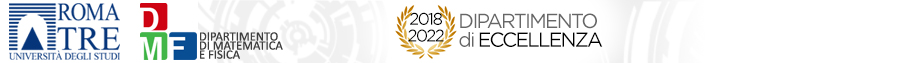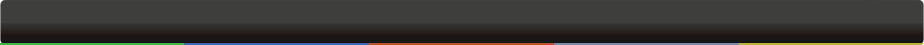# Seminari del Dipartimento

 GeometriaStable cohomology of moduli spaces of low-gonality curvesAngelina Zheng 09-02-2023 - 14:15Largo San Leonardo Murialdo, 1 The moduli space $mathcal{M}_g$ of curves of genus $g$ is a central object in algebraic geometry and our main goal is to study it from the point of view of one of the most important topological invariant, its rational cohomology. For $ggeq5,$ the rational cohomology of $mathcal{M}_g$ is still unknown and one way to approach this problem is by computing first the rational cohomology of some locus inside $mathcal{M}_g$, such as the trigonal one $mathcal{T}_g$. In the first part of the talk, we will study the rational cohomology of $mathcal{T}_g$. Specifically, we prove that, similarly to the cohomology of $mathcal{M}_g$, the cohomology ring of of $mathcal{T}_g$ stabilizes and it coincides with the tautological ring within the stable range. This will be done by studying the natural embedding of trigonal curves in Hirzebruch surfaces and by using Gorinov-Vassiliev's method for the cohomology of complements of discriminants. In the second part, we will inspect what happens for curves, which are also embedded in Hirzebruch surfaces but have gonality different from $3$. In particular I will discuss a  joint work with Jonas Bergstr"om which describes the stable cohomology of the moduli space of gonality $2$ curves, embedded on a Hirzebruch surface of fixed degree, and how this moduli space relates to the one parametrizing hyperelliptic curves with marked points. Il seminario sarà presentato in presenza nell'aula M1. Per ulteriori informazioni si può contattare gli organizzatori all'email amos.turchet@uniroma3.it.  org: Turchet Amos